It is very simple to run simple pool models in SoilR. There are a number of pool models that are available as default, but you can also implement your own version of a pool model. In this tutorial we will show you how to run a few of the pool models already available in SoilR under different assumptions of number of pools, type of C inputs, and environmental effects.

## One pool model with constant coefficients

The simplest way to describe soil carbon dynamics is a model of the form

$\frac{dC}{dt} = I - k \cdot C,$ where the change in C content in the soil over time dC/dt depents on the inputs I and a constant decomposition rate k. This model structure is implemented in SoilR with the function OnepModel.

First, open R or RStudio and load SoilR with the command

library(SoilR)


The text preceeded by ## is automatically created by R and is simply telling you that SoilR loaded additional packages. At this point you may want to look at the help of the function that runs a one-pool model by typing ?OnepModel in the R command line. You will see that this function requires four main arguments: a vector of time-points t to calculate the solution, a decomposition rate k, an initial amount of carbon C0, and the carbon inputs to the soil In. We will create each of these required arguments separately to better show the process of creating a model in SoilR.

To solve the model and run a simulation, we need to tell SoilR when to start and stop the simulations. We also need to know at what time step we want the model to run. In SoilR, the user has complete control on these arguments. The following code creates a vector of times starting at an arbitrary time 0 and ending after 10 units of time. In this case we will assume units of years, but again, the user has complete control on the time units to use; they only need to be consistent with the units of the other arguments in the model (we will return to this point later). We also will tell SoilR to run in monthly time steps.

t_start=0
t_end=10
t_step=1/12
t=seq(t_start,t_end,t_step)


Now, we need to specify the value of the decomposition rate as well as the initial amount of carbon and the inputs. Since the units of time we are using are in years, we need to be consistent with the units of the decomposition rate and the inputs. For the decomposition rate the units must be in 1/yr. The units of the initial amount of carbon must be in units of mass, and for this example we will assume units of kg. Therefore, the units for the inputs must be in kg/yr

t=seq(0,10,by=0.2)
k=0.8 # 1/time
C0=100 # mass
In = 30 # mass/time


We have now all the arguments required to run the function OnepModel. This function simply initializes the model and checks that everything is consistent. We will store the output of this function in an object with name Model1

Model1=OnepModel(t,k,C0,In)


After creating this object, we will calculate the amount of carbon predicted over time by running SoilR function getC as

Ct1=getC(Model1)


The output of the model is now stored in the object Ct1, which is the amount of carbon stored in the soil at every time step. We can plot the output with

plot(t, Ct1, type="l", ylab="Carbon stocks (kg)", xlab="Time (years)")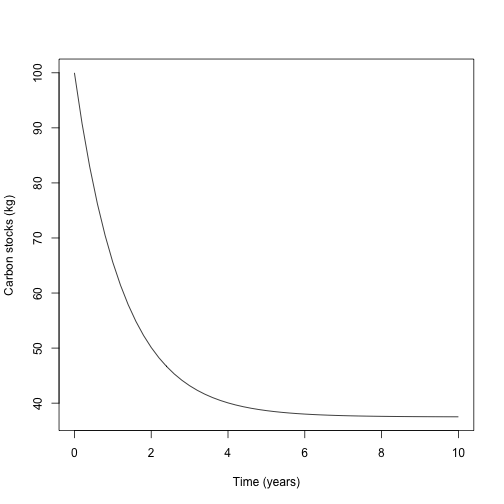In SoilR, it is also possible to calculate the amount of carbon released from the soil during the simulation either as respired C or as DOC. The function that calculates the amount of C release for each time step is getReleaseFlux. Similarly, SoilR calculates the amount of accumulated release with the function getAccumulatedRelease. Applying these functions and plotting the results we obtain

Rt1=getReleaseFlux(Model1)
Rc1=getAccumulatedRelease(Model1)

par(mfrow=c(2,1), mar=c(5,4,1,1)) # Splits the plotting area in two and adjusts margins
plot(t, Rt1, type="l", ylab="Carbon released (kg/yr)",
xlab="Time (years)")

plot(t, Rc1, type="l",ylab="Cummulative carbon released (kg)",
xlab="Time (years)")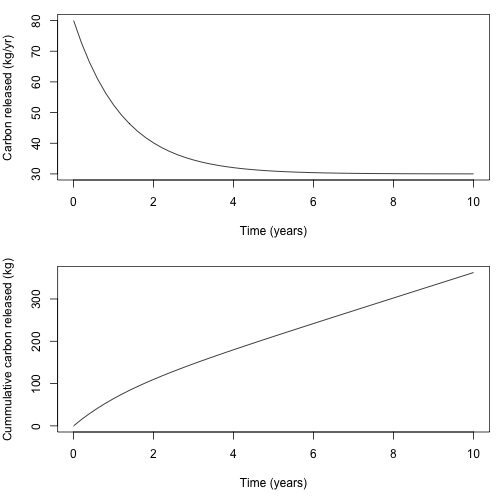## Variable inputs

The arguments in SoilR do not necessarily have to be constant, they can vary in time. For example, we can implement a one-pool model of the form $\frac{dC}{dt} = I(t) - k \cdot C$ where for each time-step the amount of C inputs changes. In this case, the argument In can be provided as an R data.frame. We can create such a data frame for this example using random numbers drawn from a normal distribution as

randomInputs=data.frame(time=t,In=rnorm(n=length(t),mean=30,sd=5))
plot(randomInputs,type="o", xlab="Time (years)", ylab="Carbon inputs (kg/yr)" )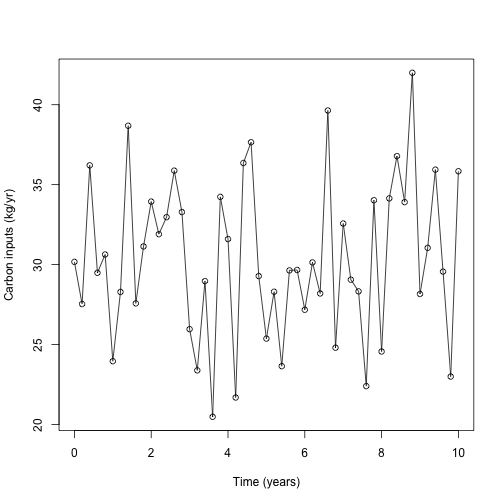We can now create a new model using these random values for the inputs and compare with the previous simulation with constant coefficients

Model2=OnepModel(t,k,C0,In=randomInputs)
Ct2=getC(Model2)
Rt2=getReleaseFlux(Model2)

par(mfrow=c(2,1),mar=c(5,4,1,1))
plot(t,Ct2,type="l",col=2,ylab="Carbon stock (kg)", xlab="Time (years)")
lines(t,Ct1)
legend("topright",c("Constant inputs","Random inputs"),col=c(1,2),lty=1,bty="n")

plot(t,Rt2,type="l",col=2,ylab="Carbon release (kg/yr)", xlab="Time (years)")
lines(t,Rt1)
legend("topright",c("Constant inputs","Random inputs"),col=c(1,2),lty=1,bty="n")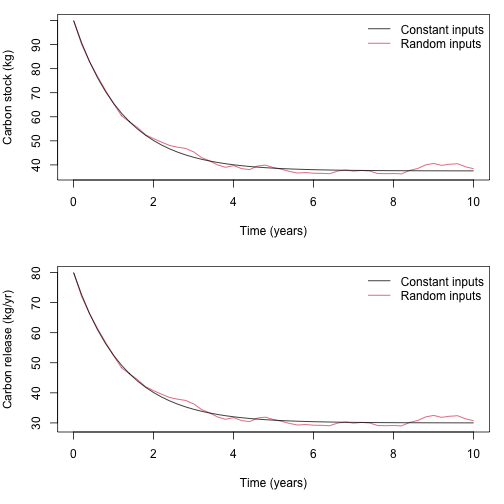## Variable environmental effects

Effects of the environment on decomposition rates are included in SoilR through an environmental modifier as $\frac{dC}{dt} = I(t) - \xi(t) \cdot k \cdot C$ where the environmental modifier is a product of different functions that depend on environmental variables as $\xi(t) = \prod_i f(X_i).$

For the purpose of this example, we will assume only a temperature effect using a Q10 function as $\xi(t) = f(T(t)) = Q_{10}^{(T(t)-10)/10}.$ with Q10 = 1.4. For this example, we will create random values of temperature for each time step.

Temp=rnorm(n=length(t),mean=10,sd=2)
xi=fT.Q10(Temp,Q10=1.4)

plot(t,xi,type="l", xlab="Time (years)", ylab=expression(xi))
abline(h=1,lty=2)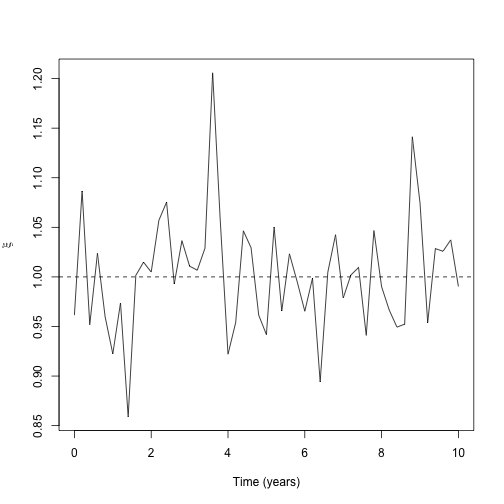In SoilR, the time-dependent abiotic effect on decomposition must be introduced as a data.frame of the form

Xi=data.frame(time=t,xi=xi)


Now we can run a model with temperature effects on decomposition rates

Model3=OnepModel(t,k,C0,In=30,xi=Xi)
Ct3=getC(Model3)
Rt3=getReleaseFlux(Model3)

par(mfrow=c(2,1), mar=c(5,4,1,1))
plot(t,Ct3,type="l",col=4,ylab="Carbon stock (kg)", xlab="Time (years)")
lines(t,Ct2,col=2)
lines(t,Ct1)
legend("topright",c("Constant inputs","Random inputs","Constant inputs, variable temperature"),col=c(1,2,4),lty=1,bty="n")

plot(t,Rt3,type="l",col=4,ylab="Carbon release (kg/yr)", xlab="Time (years)")
lines(t,Rt2,col=2)
lines(t,Rt1)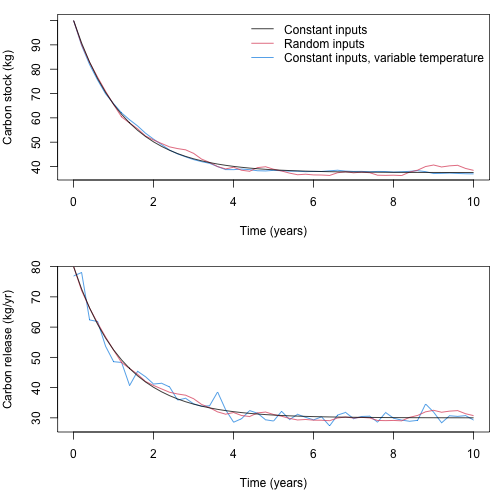## Summary

This tutorial showed how to run a simple one-pool model in SoilR. The main arguments needed to run this model are a vector of time steps t, the value of a decomposition rate k, the initial amount of carbon stored in the system at the begining of the simulation C0, the inputs to the soil In, and the environmental or abiotic effects that modify decomposition rates. You may have noticed that these simulations make no steady-state assumptions, and the C inputs to soil and the decomposition rates may change over time.

Potential applications of this model include, but are not limited, to calculation of carbon stocks or release for a variety of sites with different climatic variables, effects of changes in the inputs after land-use change on carbon stocks, effects of temperature variability on release fluxes, etc.